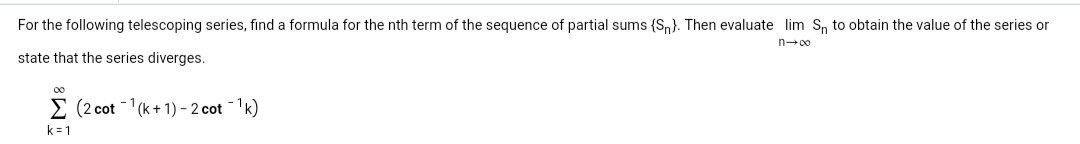# For the following telescoping series, find a formula for the nth term of the sequence of partial sums (Sn). Then evaluate lim Sn to obtain the value of the series orstate that the series diverges.n-00Σ(2 cot-1(k + 1)-2cot-k)k-1

Question
241 viewshelp_outlineImage TranscriptioncloseFor the following telescoping series, find a formula for the nth term of the sequence of partial sums (Sn). Then evaluate lim Sn to obtain the value of the series or state that the series diverges. n-00 Σ (2 cot-1(k + 1)-2cot-k) k-1 fullscreen
check_circle

Step 1

To find a formula for the nth term of the sequence of partial sums (Sn). Consider the given telescoping series and find some of the terms.

Step 2

Find the formula for the nth partial sum as follows.

Step 3

Evaluate the limit of Sn as n t...

### Want to see the full answer?

See Solution

#### Want to see this answer and more?

Solutions are written by subject experts who are available 24/7. Questions are typically answered within 1 hour.*

See Solution
*Response times may vary by subject and question.
Tagged in

### Other